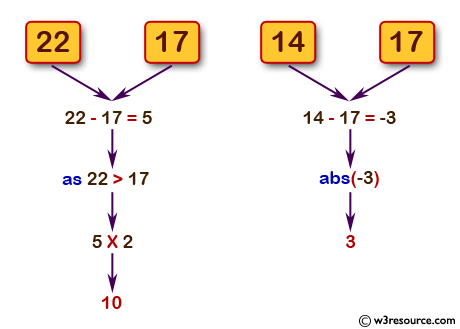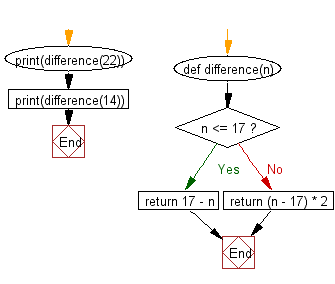﻿ Python: Get the difference between a given number and 17, if the number is greater than 17 return double the absolute difference - w3resource# Python: Get the difference between a given number and 17, if the number is greater than 17 return double the absolute difference

## Python Basic: Exercise-16 with Solution

Write a Python program to get the difference between a given number and 17, if the number is greater than 17 return double the absolute difference.

Python if-else syntax:

```if condition :
indentedStatementBlockForTrueCondition
else:
indentedStatementBlockForFalseCondition
```

These statement blocks can have any number of statements, and can include about any kind of statement.

Pictorial Presentation:Sample Solution:-

Python Code:

``````def difference(n):
if n <= 17:
return 17 - n
else:
return (n - 17) * 2

print(difference(22))
print(difference(14))
```
```

Sample Output:

```10
3
```

Flowchart:## Visualize Python code execution:

The following tool visualize what the computer is doing step-by-step as it executes the said program:

Python Code Editor:

Have another way to solve this solution? Contribute your code (and comments) through Disqus.

What is the difficulty level of this exercise?

Test your Python skills with w3resource's quiz

﻿

## Python: Tips of the Day

Try-catch-else construct:

```try:
foo()
except Exception:
print("Exception occured")
else:
print("Exception didnt occur")
finally:
print("Always gets here")
```